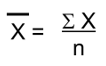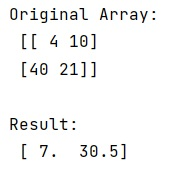# Calculate mean across dimension in a 2D array

Learn, how to calculate mean across dimension in a 2D array in Python?
Submitted by Pranit Sharma, on January 09, 2023

NumPy is an abbreviated form of Numerical Python. It is used for different types of scientific operations in python. Numpy is a vast library in python which is used for almost every kind of scientific or mathematical operation. It is itself an array which is a collection of various methods and functions for processing the arrays.

## Calculating mean across dimension in a 2D array

Mean is nothing but an average value of a series of a number. Mathematically, the mean can be calculated as:Here, x̄ is the mean, x is the summation of all the values and n is the total number of values/elements.

Suppose we have a series of numbers from 1 to 10, then the average of this series will be:

```∑x = 1+2+3+4+5+6+7+8+9+10
∑x = 55
n = 10
x̄ = 55/10
x̄ = 5.5
```

Numpy provides a method called arr.mean() which will take an argument called axis=1 or axis=0 to calculate mean across column or row respectively.

Let us understand with the help of an example,

## Python code to calculate mean across dimension in a 2D array

```# Import numpy
import numpy as np

# Creating an array
arr = np.array([[4, 10], [40, 21]])

# Display original array
print("Original Array:\n",arr,"\n")

# Calculating mean
res = arr.mean(axis=1)

# Display result
print("Result:\n",res,"\n")
```

Output: